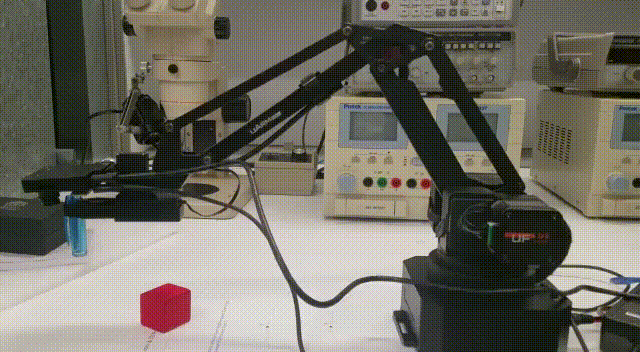# "new algorithm" from the "视觉套件使用手册"

I have two questions !!!

1. In the Arduino, there is an algorithm shown below:
//new algorithm
x_uarm = y_openmv*(-0.7035)-3.635 + 88 + 70 + 200;
y_uarm = x_openmv*(-0.7488)+12.391 + 107.5 + 15 +0;

Can someone explain more about the equation?

1. my purpose is similar to the “视觉套件使用手册”, however, I want to use the gripper instead of pump.
Now, the problem is the tracking position is not right if I change to use gripper. In the picture shown below, I want the blue pen touch the red box. Can someone tell the quickest way to solve that problem? By the way the pump is perfectly working.I would like to know this too